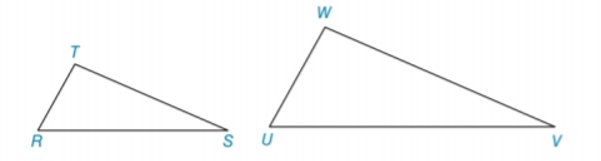Chapter 5.3, Problem 6E### Elementary Geometry for College St...

6th Edition
Daniel C. Alexander + 1 other
ISBN: 9781285195698

#### Solutions

Chapter
Section### Elementary Geometry for College St...

6th Edition
Daniel C. Alexander + 1 other
ISBN: 9781285195698
Textbook Problem
1 views

# In Exercises 5 to 8, name the method ( A A , S S S ~ ,   o r   S A S ~ ) that is used to show that the triangles are similar. ∠ T ≅ ∠ W and ∠ R ≅ ∠ UTo determine

To name:

The method that is used to show that the triangles are similar.

Explanation

Given:

The triangles,

TW and RU

Approach:

Two polygons are similar if and only if two conditions are satisfied.

1. All pairs of corresponding angles are congruent.

2. All pairs of corresponding sides are proportional.

Definitions:

AA:

If the two angles of one triangle are congruent to the two angles of another triangle, then the triangles are similar.

SAS:

If an angle of one triangle is congruent to an angle of a second triangle and the pairs of sides including the angles are proportional (in length), then the triangles are similar

### Still sussing out bartleby?

Check out a sample textbook solution.

See a sample solution

#### The Solution to Your Study Problems

Bartleby provides explanations to thousands of textbook problems written by our experts, many with advanced degrees!

Get Started

#### Given that the point P(3, 5) lies on the line kx + 3y + 9 = 0, find k.

Applied Calculus for the Managerial, Life, and Social Sciences: A Brief Approach

#### Find f. f(t) = 4/(1 + t2), f(1) = 0

Single Variable Calculus: Early Transcendentals, Volume I

#### Maximize Subject to P=3x4y x+y45x2y10x0,y0

Finite Mathematics for the Managerial, Life, and Social Sciences

#### i=1n(4i2+i)= a) 4i=1n(i2+i) b) 4i=1ni2+i=1ni c) i=1n5i2 d) 4i=1ni2+4i=1ni

Study Guide for Stewart's Single Variable Calculus: Early Transcendentals, 8th